Under:

# Extracting A_LL and DeltaG

## Determining state of beam polarization for Monte Carlo events

While Pythia does a pretty good job of simulating prompt photon production in p+p collisions, it does not include polarization for the colliding protons nor partons. A statistical method for assigning polarization states for each event based on ALL  is demonstrated in this section. For an average number of interactions for each unpolarized bunch crossing, Neff, the occurence of an event with a particular polarization state obeys a Poisson distribution with average yield of events per bunch crossing: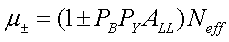For simplicity, the polarizations of the blue and yellow beams are assumed to be P

B

=P

Y

=0.7 and N

eff

=0.01. The "+" spin state defines the case where both beams have the same helicities and the "-" spin state for the case of opposite helicities. The asymmetry A

LL

is calculated from the initial states polarized and unpolarized parton distribution functions and parton-level asymmetry:The algorithm then consists in alternatively drawing a random value N

int

from the Poisson distributions with mean μ

+

and μ

-

until N

int

>0 at which point an interaction has occured and the event is assigned the current spin state. The functioning of the algorithm is illustrated in Figure 1a where an input A

LL

=0.2 was fixed and N

trials

=500 different asymmetries were calculated. Each trial integrated N

total

=300 events. It is then expected that the mean A

LL

~0.2 and the statistical precision~0.1:Indeed, both the A

LL

and its error are reproduced. In addition, variations on the number of events per trial were investigated (N

total

) in Figure 1b. The extracted width of the Gaussian distribution for A

LL

is consistent with the prediction for the error (red curve).

• ROOT macro used to generate Figure 1a SimALL.C
• ROOT macro used to generate Figure 1b SimALL2.C
 Figure 1a Figure 1b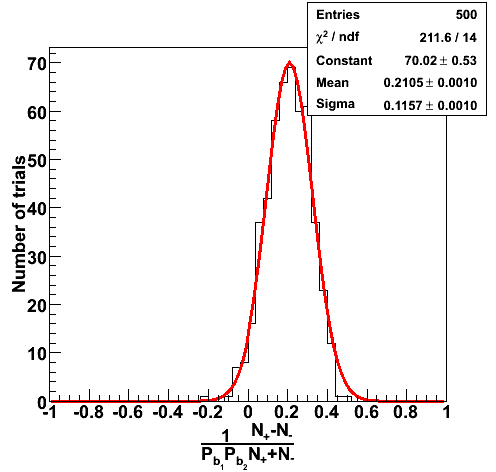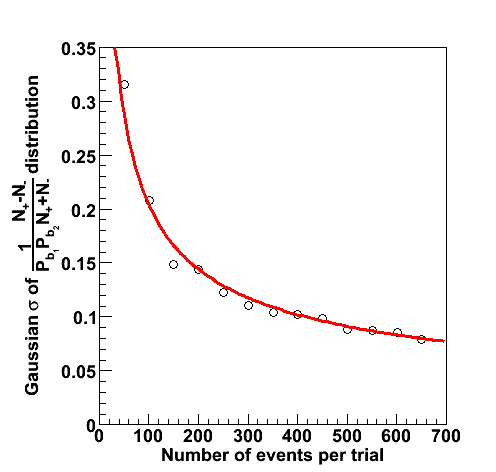## Event reconstruction

For this study, the gamma-jets Monte Carlo sample for all partonic pT were used. As an example, the prompt photon processes for the partonic pT bin 9-11 GeV and their total cross sections are listed in the table below. Each partonic pT bin was divided into 15 files each of 2000 events.

``` ==============================================================================
I                                  I                            I            I
I            Subprocess            I      Number of points      I    Sigma   I
I                                  I                            I            I
I----------------------------------I----------------------------I    (mb)    I
I                                  I                            I            I
I N:o Type                         I    Generated         Tried I            I
I                                  I                            I            I
==============================================================================
I                                  I                            I            I
I   0 All included subprocesses    I         2000          9365 I  3.074E-06 I
I  14 f + fbar -> g + gamma        I          331          1337 I  4.930E-07 I
I  18 f + fbar -> gamma + gamma    I            2             8 I  1.941E-09 I
I  29 f + g -> f + gamma           I         1667          8019 I  2.579E-06 I
I 114 g + g -> gamma + gamma       I            0             1 I  1.191E-10 I
I 115 g + g -> g + gamma           I            0             0 I  0.000E+00 I
I                                  I                            I            I
==============================================================================
```

The cross sections for the different partonic pT bins has been tabulated by Michael Betancourt and is reproduced here for convenience.

 Partonic pT [GeV] Cross Section [mb] 3-4 0.0002962 4-5 0.0000891 5-7 0.0000494 7-9 0.0000110 9-11 0.00000314 11-15 0.00000149 15-25 0.000000317 25-35 0.00000000990 35-45 0.000000000449

These events were processed through the 2006 pp200 analysis chain, albeit without any cuts on the SMD. The simulated quantities were taken from the Pythia record and the reconstructed ones from the analysis.

 Figure 2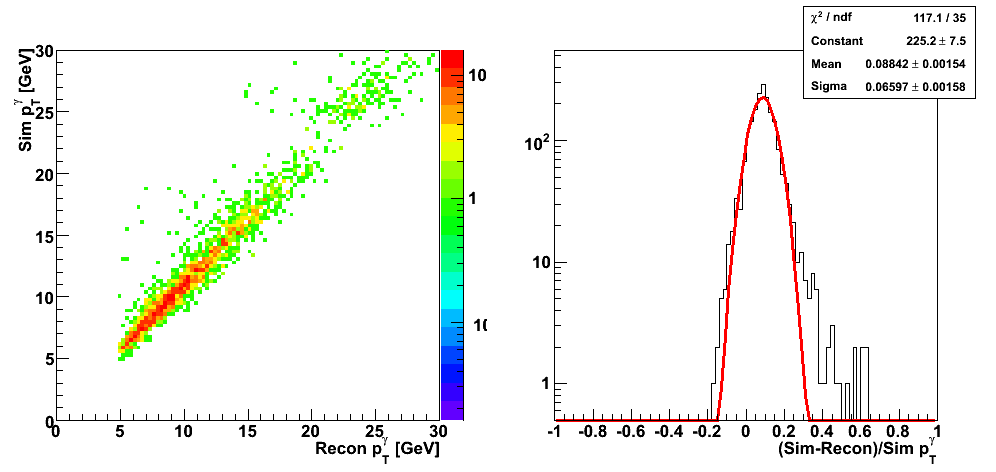Figure 3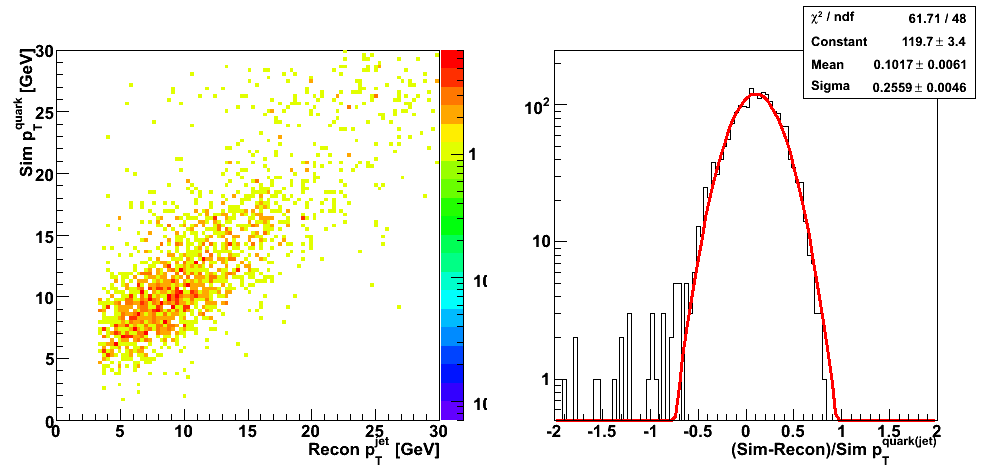Figure 4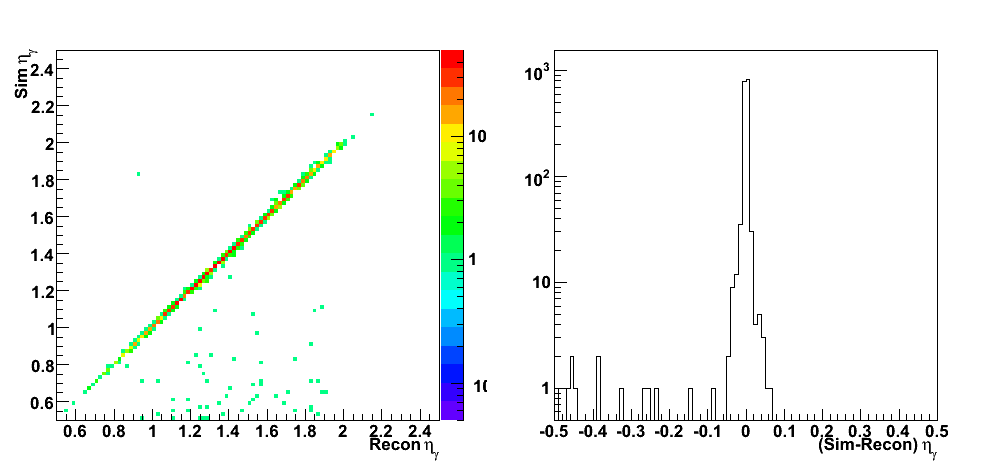Figure 5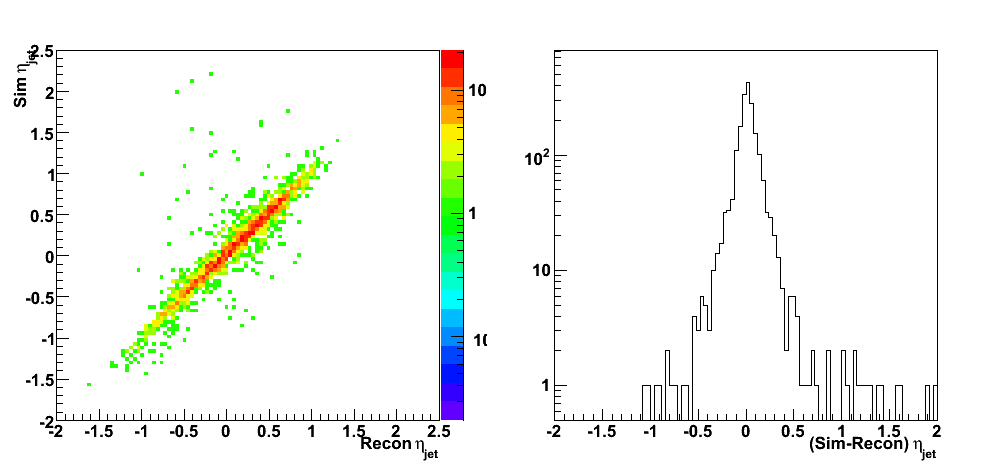Figure 6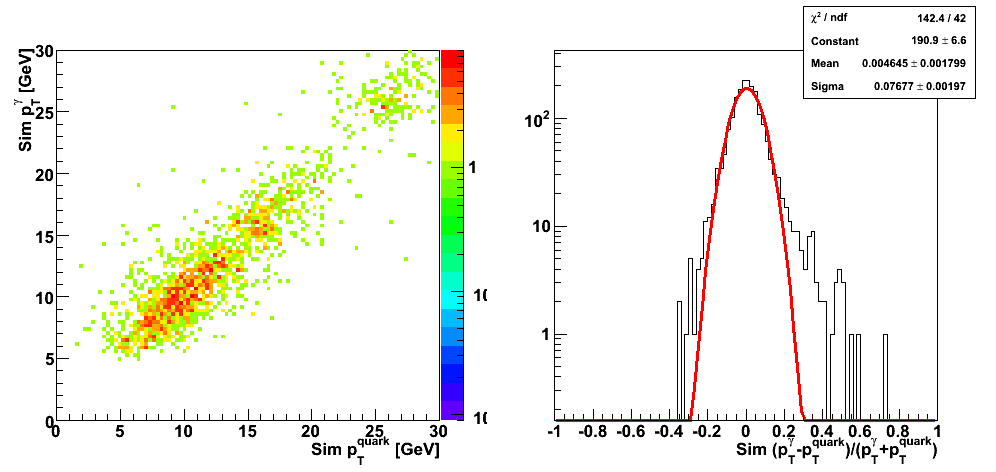Figure 7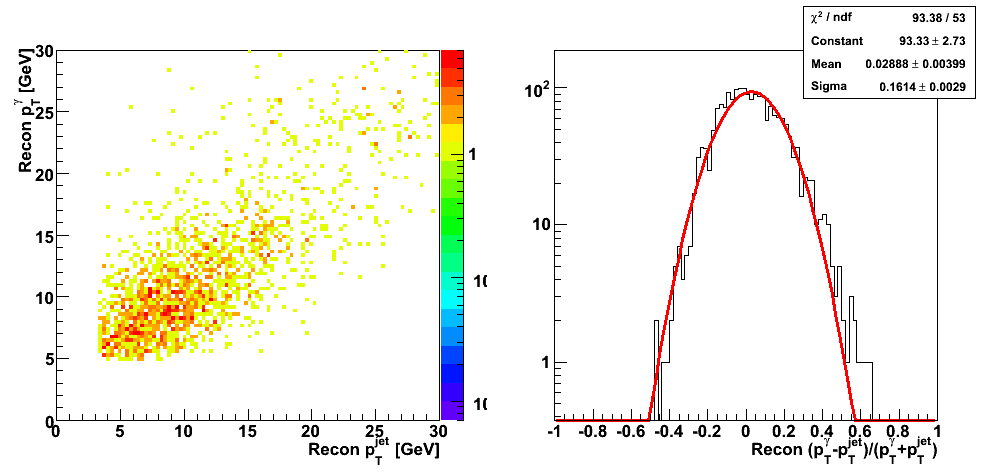Figure 8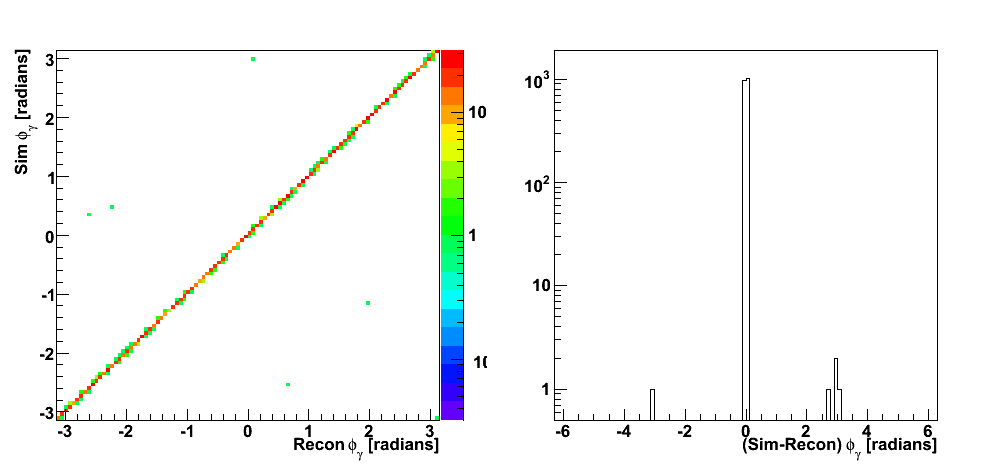Figure 9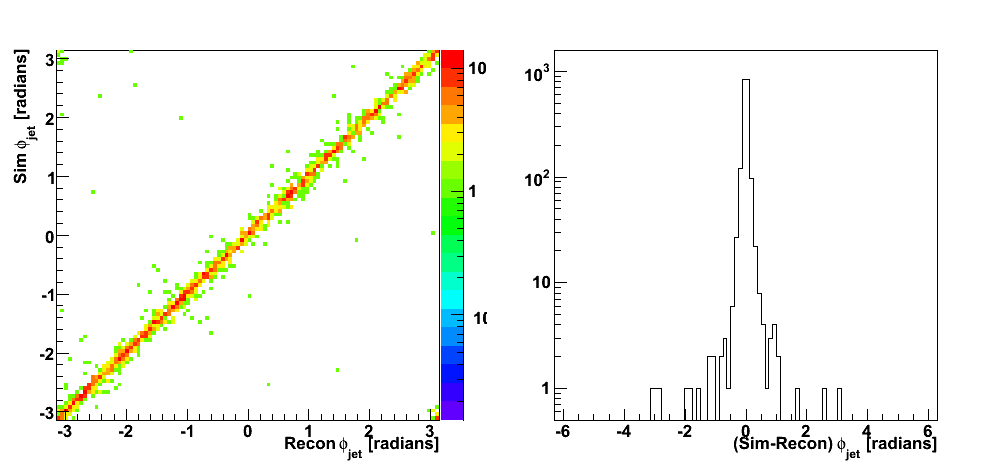## Partonic kinematics reconstruction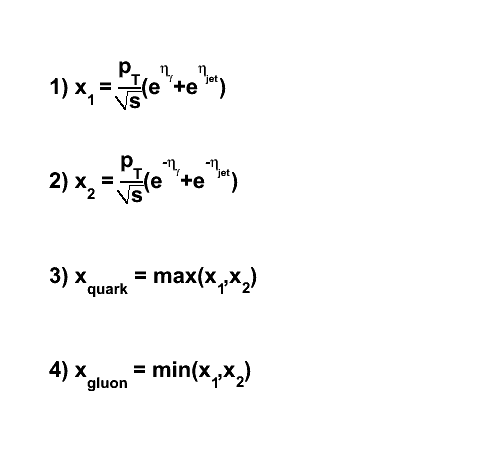PartonicKinematics.C
 Figure 10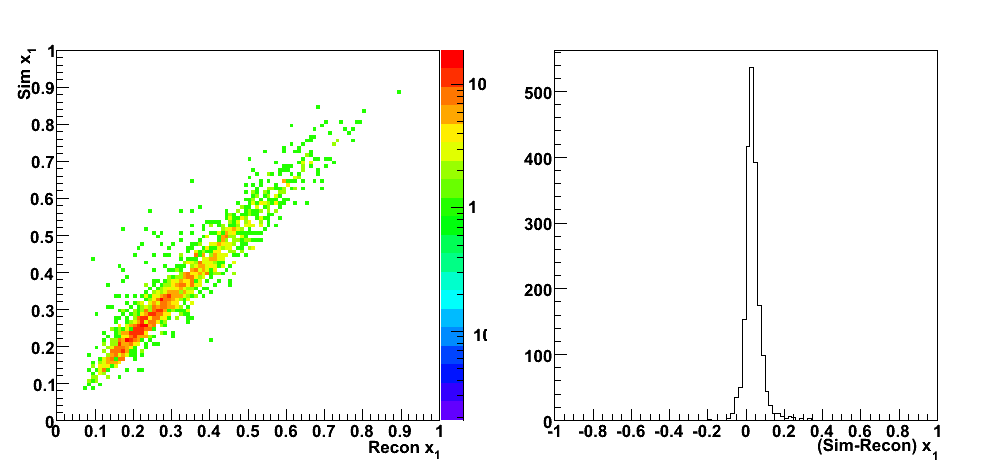Figure 11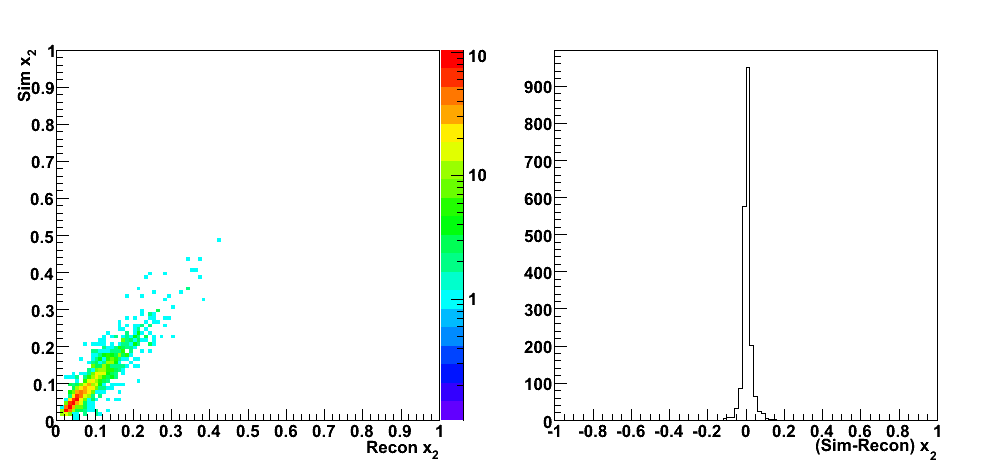Figure 12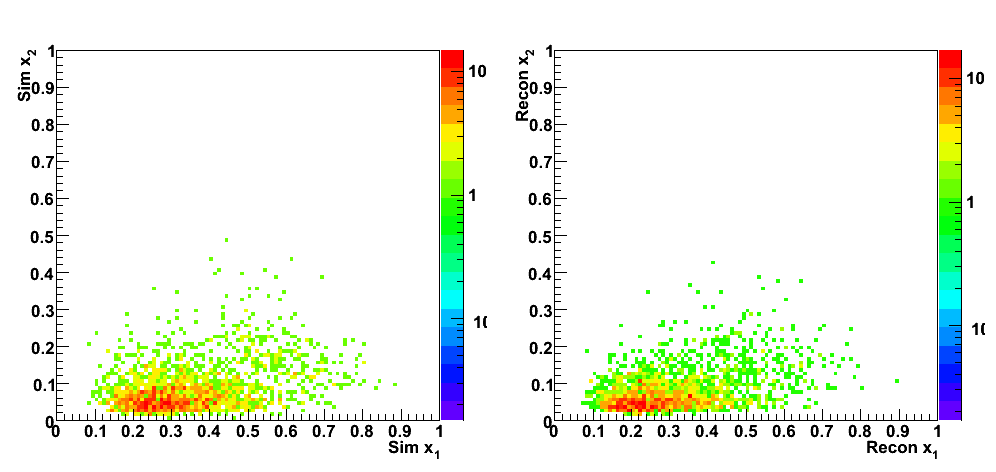Figure 13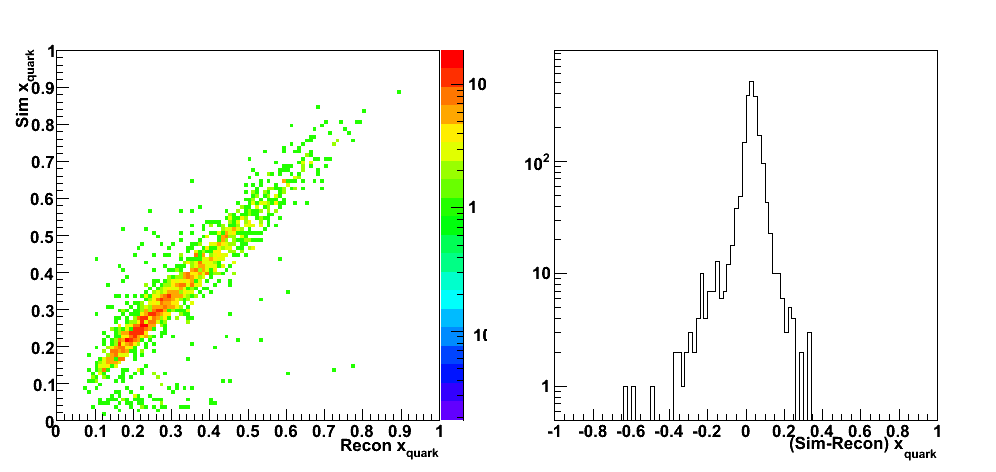Figure 14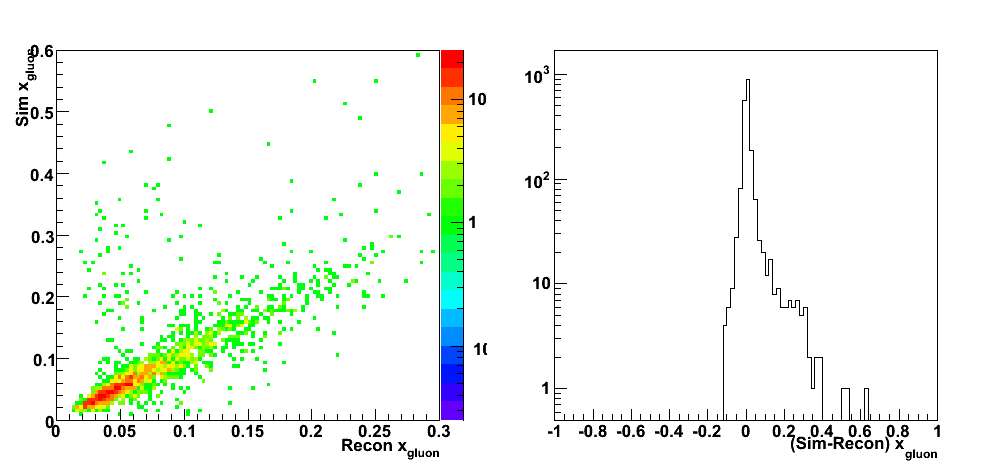Figure 15 (a)Figure 15 (b): quark from proton 1 (blue beam, +z direction)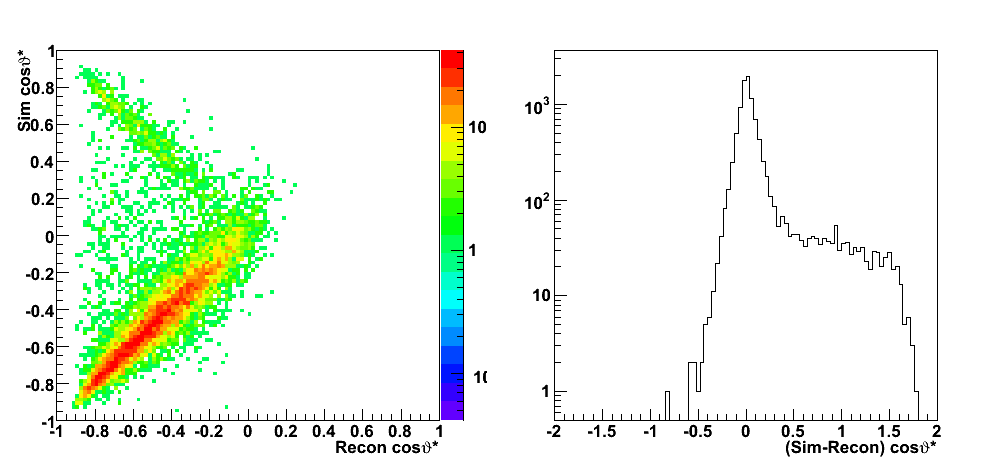Figure 15 (c): quark from proton 1 (blue beam, +z direction) and q + g -> q + gamma subprocess (29)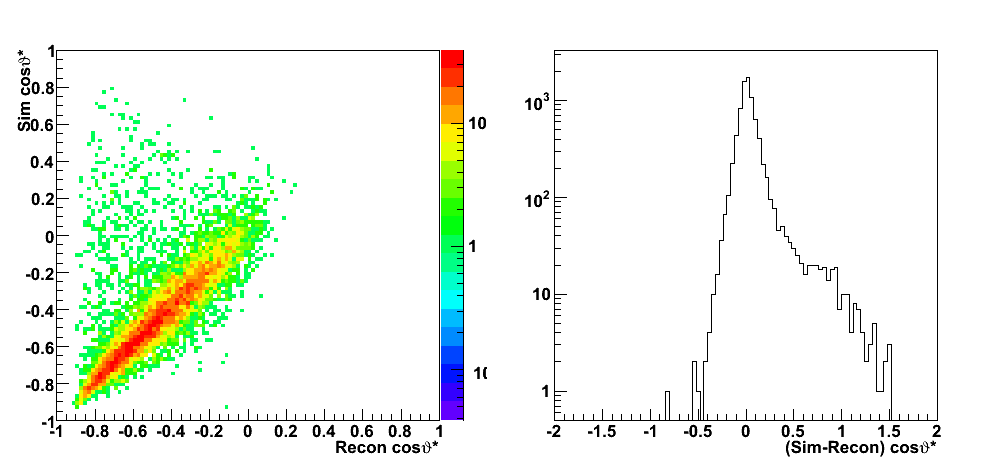Figure 15 (d): quark from proton 1 (blue beam, +z direction) and q + qbar -> gamma + g subprocess (14)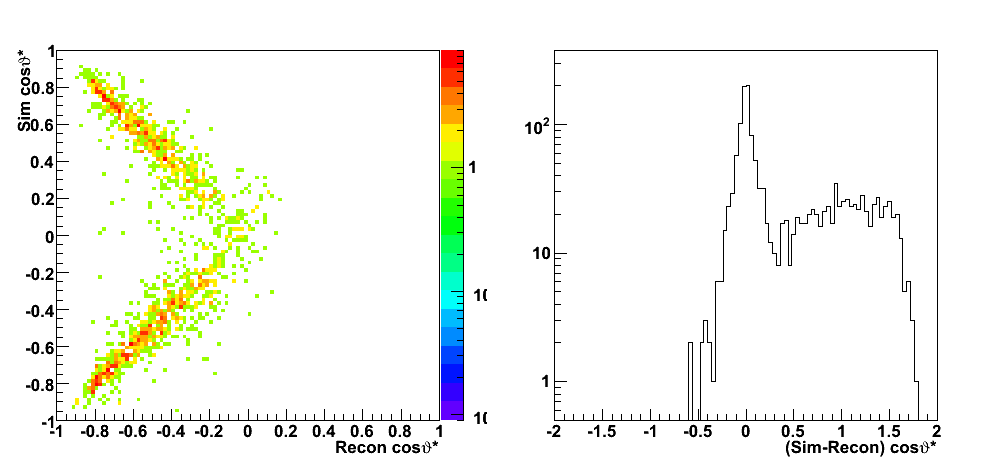## Predictions for A_LL and direct determination of DeltaG(x)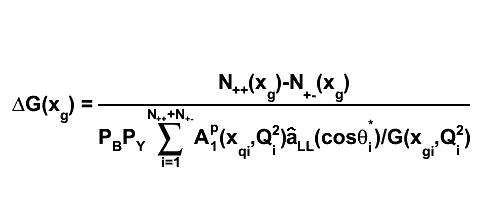DeltaG.C
 Figure 16 (a)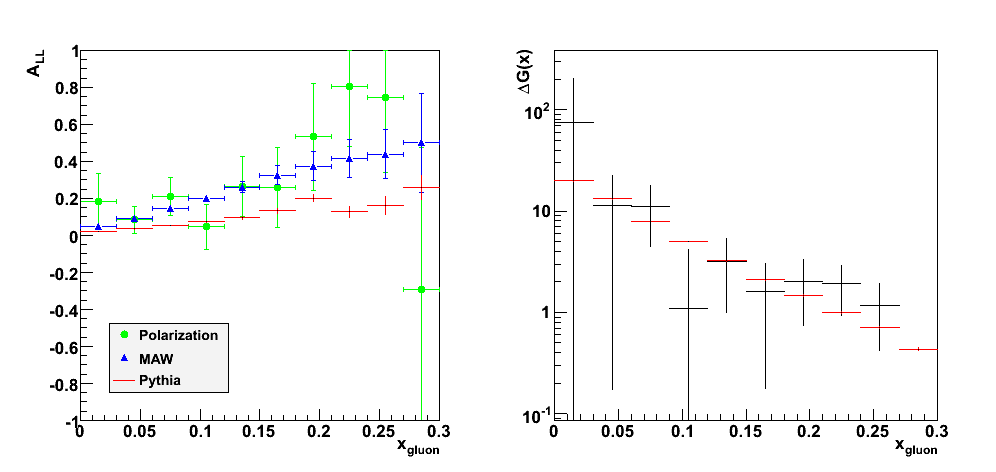Figure 16 (b): quark from proton 1 (blue beam, +z direction)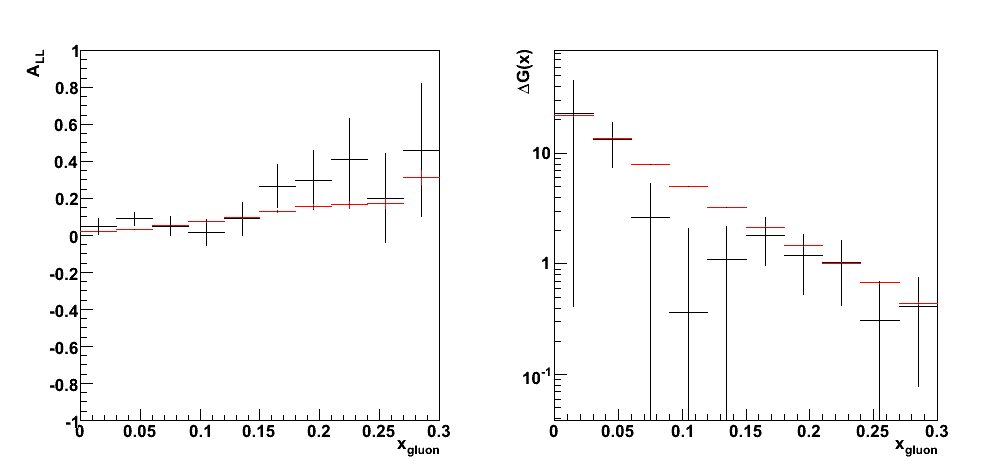Figure 16 (c): quark from proton 1 (blue beam, +z direction) and q + g -> q + gamma subprocess (29)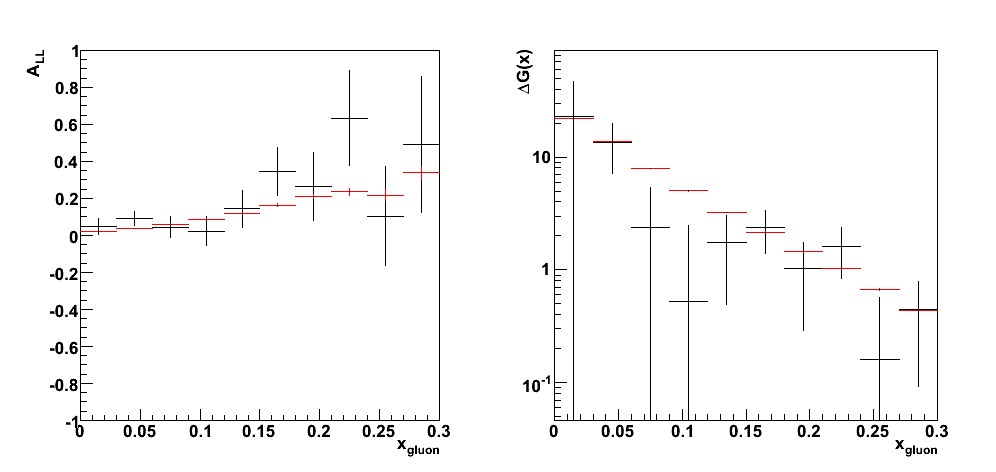Figure 16 (d): quark from proton 1 (blue beam, +z direction) and q + qbar -> gamma + g subprocess (14)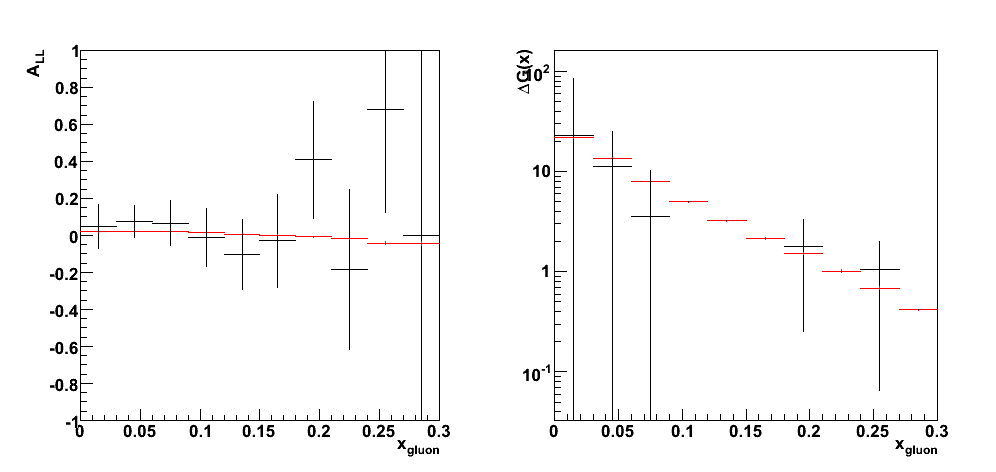## References

Pibero Djawotho
Last updated Wed Jul 16 10:29:22 EDT 2008